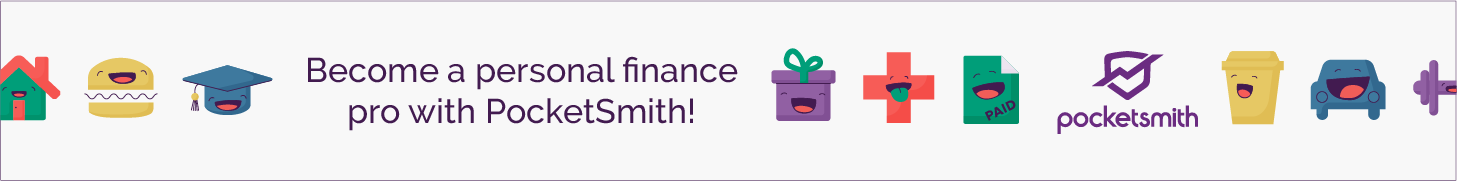# The Most Important Thing You Will Ever Learn: Part Two

Now earlier, I wrote a post about the most important thing that you will ever learn. If you didn’t read it, I would recommend that you go back and read it. It will make this post make more sense.

To really hit home the power of exponential growth let’s look at a simple example.  Think of a game of chess. The game of chess was said to have been invented by a mathematician who worked for a king. The king was happy with the game that the mathematician had created. The king wanted to reward the mathematician.

The mathematician said that his needs were modest. He said, please place one grain of wheat on the first square of the board, and then double the amount of wheat on the next board, which is two grains of wheat. Double this again for the next square for a total of four grains, and so on until each square on the cheese board had grains of wheat. This would be enough payment for the mathematician

The king thought that the mathematician was a fool. The king was ready to give the mathematician a real reward, and all he asked was for a few grains of wheat!

So there are 8 grains of wheat on the fourth square. This number comes from multiplying three twos, 2 times 2 times 2, one “two” less than the total squares. To calculate the number of wheat on the 64th square, we need to multiply 63 twos together.

1 square = 1 grain

2 squares = 2 grains + 1 grains = 3 total

3 squares = 4 grains + 3 grains = 7 total

4 squares = 8 grains + 7 grains = 15 total

Look at the way the totals build-up, when we have one grain on the first square the total on the board is 1. Then we add two grains that make a total three. Then we put on four grains on square 3, now the total is seven. Seven is a grain less than eight, it’s a grain less than three “two”s multiplied together. The next total 15 is a grain less than four “two”s multiplied together.

This rule continues in each case, so when we are done, the total number of grains is one less than the number I get multiply 64 “two”s together. Now the question is, how big of a pile of wheat is this?

Would it be a pile big enough to fill the room you are sitting in? To fill your entire house? How much wheat are we talking?

The answer is that it is roughly 300 times last years worldwide harvest.  It could even possibly be more wheat that humans have ever been harvested for the entire history of the world.

How did the number get soooo big?

We are steadily growing the number of grains until we have double the original by a total 63 times.

Now the important thing to learn is that the total you have is larger than the total of all previous doubling times in any doubling time. For example, 8 grains of wheat on the 8th square are larger than the total of square 1, 2 and 3 combined, 7.

When we put thirty-two grains on the six square, the 32 is larger than the total of 31 that were already there. Every time the growing quantity doubles, it is larger than all the total quantity of all preceding doubling periods.

### When you really understand this point, it will blow your mind.

To give you an idea of this point, let’s look at energy consumption.  Energy consumption from the 1970s has been growing steadily around the world. The world’s energy consumption doubles every 10 to 12 years.  This is a growth rate of around 7%

Now the growth rate has slowed down in recent decades. But for the example, let’s assume that it is expected to keep growing. Now, this is a similar example to the cheeseboard.

So in 10 to 12 years, the worldwide energy consumption will double.  What does this actually mean?

What this actually means is that the worldwide consumption of energy for the year ending the decade will be greater than all the electrical energy that has ever been consumed in the entire preceding history. Think about that!

Now did you realise that this could happen from only growing at 7% over 10 years? It is incredible that in just 10 years, the world would use more energy than what has been used in all preceding history!

That is what 7% yearly growth actually means!

## Subscribe For the Latest Content!

Subscribe to Passive Income NZ — get ahead with the latest post emails directly to your inbox. As a bonus, I'll send you a FREE Personal Finance Resource Kit, so you can start your Journey to Finanical Freedom.Visit my Resources Page to find out how you can get 50% off Pocketsmith!Use This Link to get 1 month on any new car insurance policy

Information presented on the Website is intended for informational and entertainment purposes only and is not meant to be taken as financial advice. Some of the links above are affiliate links, meaning, at no additional cost to you, I will earn a commission if you click through. Please note that I only recommend products and services that I have personally used.